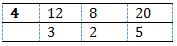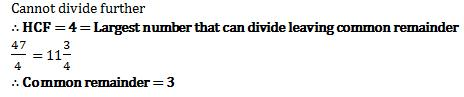Courses

# Test: LCM & GCD - 2

## 20 Questions MCQ Test Quantitative Aptitude for GMAT | Test: LCM & GCD - 2

Description
This mock test of Test: LCM & GCD - 2 for GMAT helps you for every GMAT entrance exam. This contains 20 Multiple Choice Questions for GMAT Test: LCM & GCD - 2 (mcq) to study with solutions a complete question bank. The solved questions answers in this Test: LCM & GCD - 2 quiz give you a good mix of easy questions and tough questions. GMAT students definitely take this Test: LCM & GCD - 2 exercise for a better result in the exam. You can find other Test: LCM & GCD - 2 extra questions, long questions & short questions for GMAT on EduRev as well by searching above.
QUESTION: 1

### Greatest Common Divisor of two numbers is 8 while their Least Common Multiple is 144. Find the other number if one number is 16.

Solution: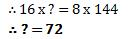QUESTION: 2

### LCM of two numbers is 138. But their GCD is 23. The numbers are in a ratio 1:6. Which is the largest number amongst the two?

Solution: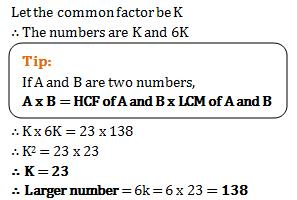QUESTION: 3

### The least common multiple of two numbers is 168 and highest common factor of them is 12. If the difference between the numbers is 60, what is the sum of the numbers?

Solution: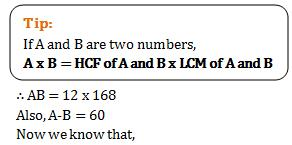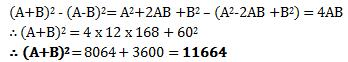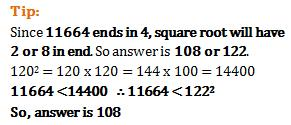QUESTION: 4

If least common multiple of two numbers is 225 and the highest common factor is 5 then find the numbers when one of the numbers is 25?

Solution: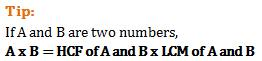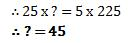QUESTION: 5

The greatest number of four digits which is divisible by 15, 25, 40, 75 is

Solution:

We want 4 digit number, so option A is not the answer
Now we want greatest number. So out of remaining options, 9600 is greatest
9600 is divisible by 25, 75, 40 and 15
So answer is C i.e. 9600

QUESTION: 6

What is the least number which when divided by the numbers 3, 5, 6, 8, 10 and 12 leaves in each case a remainder 2 but which when divided by 13 leaves no remainder?

Solution:

Approach 1 - Direct Observation

The number must be divisible by 13.
Option a, b. d are divisible. C is not.
Now the number leaves remainder 2 when divided by 3, 5, 6, 8, 10, and 12.
So 'Number -r should be divisible by 3, 5, 4 8, 10, and 12
Take Option A i.e. 312-2 = 310.
But 310 is not divisible by 3
Take Option D i.e. 1586-2 = 1584
We see that 1584 is not divisibleby 10

QUESTION: 7

When a number is divided by 893 the remainder is 193. What will be the remainder when it is divided by 47?

Solution:

In such cases and sums, simply follow these easy steps
Number is divided by 893. Remainder =193.
Also, we observe that 893 is exactly divisible by 47.
So now simply divide the remainder by 47.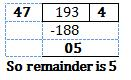QUESTION: 8

The sum of two numbers is 156 and their HCF is 13. The numbers of such number pairs is

Solution:

Highest Common Factor (HCF) is 13, that means
the numbers are multiples of 13.
We need sum of numbers to be 156.
In such sums, first write multiples of 13 till 156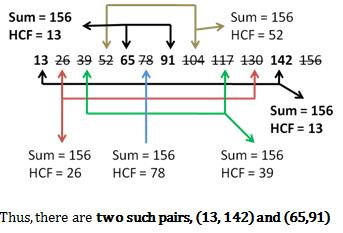QUESTION: 9

The L.C.M. of two numbers is 14560 and their H.C.F. is 13. If one of them is 416, the other is

Solution: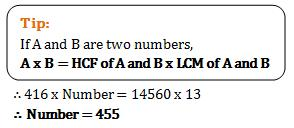QUESTION: 10

The greatest length of the scale that can measure exactly 30 cm, 90 cm, 1 m 20 cm and 1 m 35 cm lengths is

Solution:

Here we need to exactly measure these lengths.
Also, lm = 100 cm; ... 1m 35cm = 135cm and 1m 20cm = 120cm
Option 1- 5 cm can measure 30cm, 90cm, 1m 20cm and 1m 35 cm
Option 2 - 10 cm cannot measure 1m 35cm i.e. 135cm
Option 3 -15 cm can measure30cm, 90cm, 1m 20cm and 1m 35 cm
Option 4 -30 cm cannot measure 1m 35cm
Since we want greatest length 15 > 5

QUESTION: 11

What will be least number of marbles with Rohit if he can arrange them in rows of 18, 10 and 15 marbles each as well as make a perfect solid square out of all the marbles?

Solution: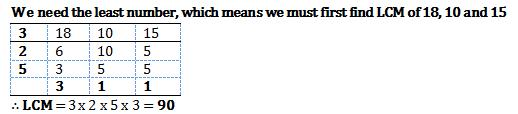90 is not a perfect square.
90=3x3x2x5
Here there are two 3's (this gives square of 3) but only one 2 and one 5
if we multiply by 2 and 5 then we get, 3 x3x 2x2x5x 5= 900
900 is perfect square = number of marbles

QUESTION: 12

3 birds fly along the circumference of a jungle. They complete one round in 27 minutes, 45 minutes and 63 minutes respectively. Since they start together, when will they meet again at the starting position?

Solution: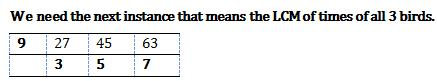∴ LCM = 9x3x5x7 = 945  =  They meet after 945 minutes.

QUESTION: 13

What least possible 4-digit number, when divided by 12, 16, 18 and 20 leaves 21 as remainder?

Solution:

Here least possible 4 digit number is needed that means we need the LCM We must first find LCM of 12, 16, 18 and 20 .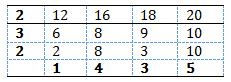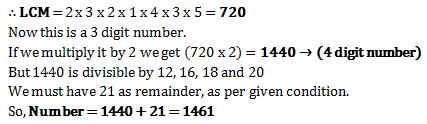QUESTION: 14

5 clocks ring automatically at intervals of 12 minutes, 8 minutes, 3 minutes, 4 minutes and 10 minutes, respectively. In 8 hours from the moment they start, how many times will they ring together?

Solution:

We need the next instances when the alarm rings.
That means the Least Common Multiple (LCM) of 3, 4, 8, 10, 12 3, 4 divide 12 so neglect them.
LCM of 8, 10 and 12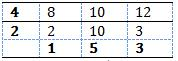∴ LCM = 4x2x5x3= 120 = They ring after 2 hours
After starting, they ring together ist time in 2 hours.
Then 2nd time in 2+2 = 4 hours.
Then 3rd time in 6 hours.
And 4th time in 8 hours.

QUESTION: 15

What least number when divided by 36, 24 and 16 leaves 11 as remainder in each case?

Solution:

Here least number is needed that means we need the LCM
We must first find LCM of 36, 24 and 16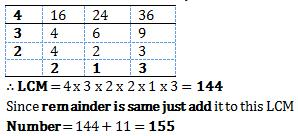QUESTION: 16

If (x - 4) is the HCF of x2 - 8x + 15 and x2 - kx +1 , then what is k?

Solution:

(x-4) is HCF i.e. a factor of both equations
∴ The equations must get satisfied for x=4
Also, when x=4, both equations are equal in value.
Putting x=4 in both equations
42 - 8(4) + 15 = 42- k(4) +1

∴ 31-32 = 17- 4k
∴ k=4

QUESTION: 17

What least number when divided by 20, 48 and 36 leaves the remainders 13, 41 and 29, respectively?

Solution:

Here least number is needed that means we need
the Least Common Multiple i.e. LCM
We must now first find LCM of 20, 36 and 48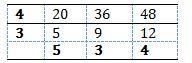:.LCM=4x3 x5 x 3 x 4 = 720
But this is not the mnverbecause there are remainders as per the given condition.
If we observe dosely, the difference between the given numbers and remainders is same
20-13 = 7; 48-1 = 7; 36-29 = 7
Difference is same = 7
So simply subtract this difference from LCM.
Number = 720 - 7 = 713

QUESTION: 18

There are 3 equilateral triangles with sides 114cm, 76cm and 152 cm. What maximum size scale can measure them exactly?

Solution: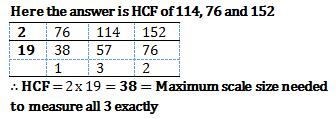QUESTION: 19

What greatest number divides 17, 42 and 93 and leaves remainders 4, 3 and 15, respectively?

Solution:

Here greatest number that can divide means the HCF
Remainders are different so simply subtract remainders from numbers
17-4 = 13; 42-3 = 39; 93-15 = 78
Now let's find HCF of 13, 39 and 78
By direct observation we can see that all numbers are divisible by 13.
∴ HCF =13 = required greatest number

QUESTION: 20

What largest number will divide 47, 35 and 27 leaving same remainder in each case? What will be the common remainder?

Solution:

What to do when we don't lcnow the remainder and the largest number which divides?
In such cases subtract the 3 numbers from each other
47-35 = 12
35-27 = 8
27-47 = -20 (Ignore minus sign)
Largest number is needed that means we need HCF of 12, 8 and 20